## Error Correcting Codes

#### Activity 1: A Blurred Message

One day, Sarah receives the note below from her friend, but it has gotten wet and some of the letters are blurred. (Blurred letters are symbolized by *.) Can you help her figure out what the letter says?

Hey!
I'm sor*y to hear *ou are sick. H*re is the mat* as*ignmen* fr*m tod*y: pg. *84 #2, *, 7, 9a, 1*, and 15. We h*d a f*re drill, but yo* didn't miss *nything...
G*t well s**n!
Ali*a

Which parts were easy to decipher? Which parts were hard?

(Hint: Click here to see the full letter to compare to what you deciphered).

You were probably able to figure out most of the text (if not all the numbers). This is because English is redundant, or has redundancy. Therefore, if there are errors (blurred letters) in an English sentence, our brains can make use of this redundancy to guess what the correct letters should be.

The redundancy in English also explains why we can use short forms when writing to our friends, particularly when writing notes or instant messaging. For example, English does not have many phrases in which the words start with an 'l', then an 'o', then another 'l', so we can easily remember that 'lol' stands for 'laughing out loud'. It is easy to understand why we want to do this - 'lol' is almost nine times shorter than 'laughing out loud'!

Now think about when you listen to a static-y radio station in the car. If there is not too much static you can understand what the announcer is saying, even if you can't quite hear all the words. Engineers call this static noise. The redundancy in English enables us to understand, or receive, the broadcaster's message, even if noise is introduced after the message is sent, or transmitted. Being able to understand messages even if they have been blurred is exactly what an error-correcting code does.

One of the first uses of error-correcting codes was in space probes. Suppose a space probe is orbiting Saturn, and wants to send data back to Earth. The probe uses radio waves to communicate with Earth, so you can imagine that there is a lot of static, or noise, that affects the message before it reaches the receiver. Also, it takes many hours for the data to reach Earth, so scientists do not want to ask the probe to retransmit its data when the noise causes errors. Instead, the scientists want to be able to understand all the data the first time it is transmitted. To ensure that the scientists can understand the message, even if errors are introduced by the noise, the probe must add redundancy to the data. The redundancy is important: in the letter above, it was much easier to understand the English (which had a lot of redundancy) than the list of math problems (where every digit was important and there was no redundancy). So, the probe must add redundancy to the data using an error-correcting code before it transmits. The scientists receiving the transmission can work backwards, using the structure introduced by the error-correcting code, to determine where noise caused errors in the data, and then correct them.

Error-correcting codes are used in many other applications besides space probes. Some examples are:

1. CD and DVD players, to correct errors made by scratches on the CD or DVD
2. cellphones
3. hard drives
4. satellites

#### Activity 2: Detecting Forged Drivers' Licenses

For some applications, we do not need to correct errors, but only detect them. For example, since it is easy for people to mistype numbers, it would be helpful if a computer could determine when the sales clerk has entered a credit card number incorrectly, or when the scanner did not read the bar code correctly. In these cases, it is easier to ask the person to re-enter the number or re-swipe the purchase, than to try to correct the error. In such cases, we just need an error-detecting code. An example of an error-detecting code is a checksum, or check digit.

Check sums are used as error-detecting codes in the following applications:

1. credit card numbers
2. social security numbers
4. ISBN, an identification number for books
5. bar codes
6. Vehicle Identification Numbers

Imagine that you are a detective at a police station in Seattle, Washington. One day, your supervisor tells you that a stolen car was recovered, and there were several Washington driver licenses in a plastic bag in the glove compartment. She asks you to investigate these driver licenses. Here are pictures of the four licenses.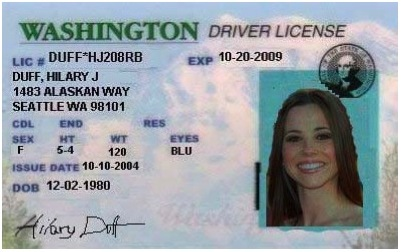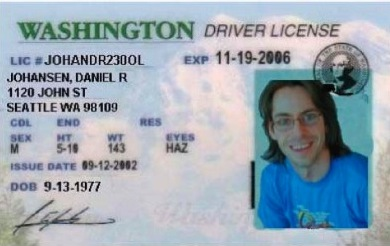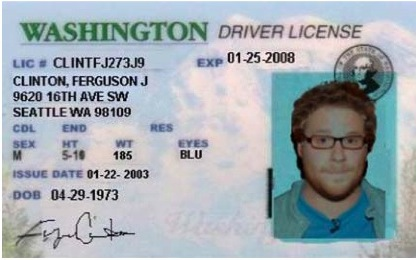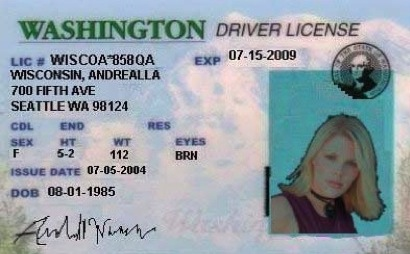How would you go about figuring out if any of these driver licenses are fake?

One plan you might have is to look up each of the licenses in the police computer. Let's pretend that the computer database for driver licenses is not working today. However, you know that the license number has a special format. You want to use this special format to find the fake license numbers.

A Washington driver license number is 12 characters long, and has the following format: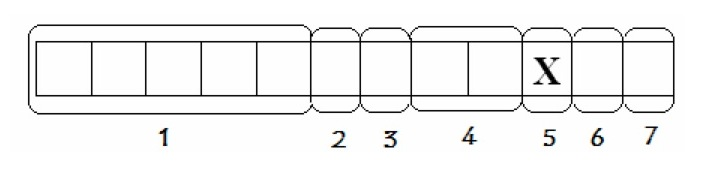where

1. Represents the first five letters of the last name. If the last name is shorter than five letters, * is used for the remaining spots.
2. Represents the first initial.
3. Represents the middle initial. * is used if there is no middle name.
4. Represents the year of birth encoded: It is 100 minus the last two digits of the year of birth.
5. The checksum: See explanation below.
6. Represents the month of birth encoded using Table 1. Use the first column unless it would result in a duplicate license.
7. Represents the day of birth, encoded as a letter or number using Table 2.

X is a checksum calculated using the rest of the license number. To get X, first convert all letters in the license number into digits, using Table 3. Next, subtract the second digit from the first, add the third digit to the answer, subtract the fourth digit from this answer, etc. If this answer is positive, X is the last digit. If the answer is negative, add 10 until you have a positive number, which is X.

Let's start with an example, and then get to the detective work. Imagine that Anita Katherine LaFrance, born October 25, 1982, comes to your office and wants a driver license number. We first encode her name and date of birth.

L A F R A A K 1 8 X P 5

Next, we convert all the letters in the license numbers into digits, using Table 3. These digits will be used to compute the checksum.

L A F R A A K 1 8 X P 5 → 3 1 6 9 1 1 2 1 8 7 5

The checksum, X, is computed by subtracting the second digit from the first, then adding the third digit to the answer, then subtracting the fourth digit from the answer, etc.

3 - 1 + 6 - 9 + 1 - 1 + 2 - 1 + 8 - 7 + 5 = 6. So, X = 6.

Putting all of this together, we get that the final driver license number for Anita Katherine LaFrance, born October 25, 1982 is

L A F R A A K 1 8 6 P 5

Now, let's get back to the mystery of the forged driver licenses. The seized driver licenses are pictured below. Using the information that you know about how the driver licenses are made, can you determine which driver licenses are forgeries and which might be real?You have just seen an example of an error-detecting code! We could tell which driver licenses were forgeries because they didn't have the right format. The next activity introduces more complicated codes. Instead of simply detecting an error, these codes actually allow the message receiver to correct some errors.

#### Activity 3: Sending Secret Messages

Remember that you are a police detective. You have figured out which driver licenses are forged. You want to leave a message for your supervisor with the name from one of the forged licenses. But, there are some mischievous people in the office who might want to pull a prank with the message. So, you decide to write the names in code. It is important that even if the pranksters change a few of the letters in your code, your supervisor will still be able to decipher the name.

Can you invent such a code?

Here is one example of a code that does what we want -- an error-correcting code using syndromes. We use the encoder sheets to convert each letter of the code to a pattern of 0s and 1s. Here is how to use the encoder sheets:

• Write down the first letter you want to encode in cell (a).
• Write does the binary pattern (0s and 1s) corresponding to this letter from the Conversion Table in the column marked (b).
• Each time there is a 1 in column (b), circle all the 1s in the row beside it.
• In each cell of row (d), write the number of circled 1s above it.
• In each cell of row (e), write 0 if the number above it is even (or zero), and 1 if it is odd.
• Row (e) becomes the code word for the letter in cell (a).

Let's start with an example. We will encode the message Hello!. Remember that we need to encode one letter at a time. Looking up in the Conversion Table, we see that 'H' corresponds to 00111. These bits go in column (b). Then, we circle the 1s in the third, fourth, and fifth rows of (c) -- next to 1s in column (b). We count up the number of circled 1s in each column, and write the total in (d). We write a 0 in the first, second, sixth, seventh and eighth columns of row (e) because the numbers in (d) of those columns are even. The numbers in (d) in the third, fourth, fifth, and ninth columns are odd so we write a 1 in row (e) for those columns. So, we get that the codeword for 'H' is 001110001.

The filled in encoder table for each of the letters 'H', 'E', 'L', 'O', '!' is below for your reference. Putting all the information from the encoders together, we see that the codeword for Hello! is
001110001  001000110  010111101  010111101  011100010  111011001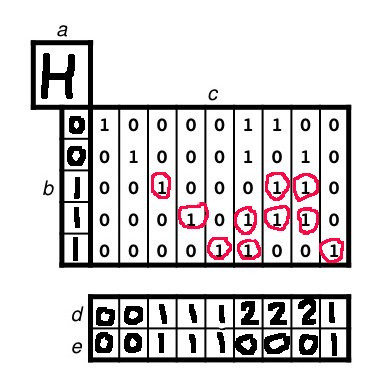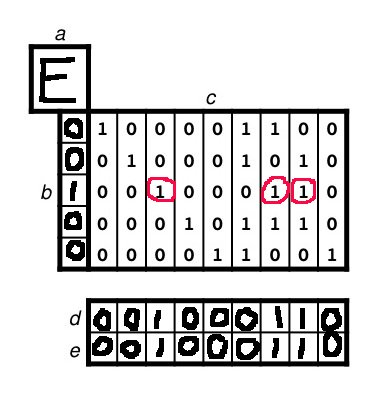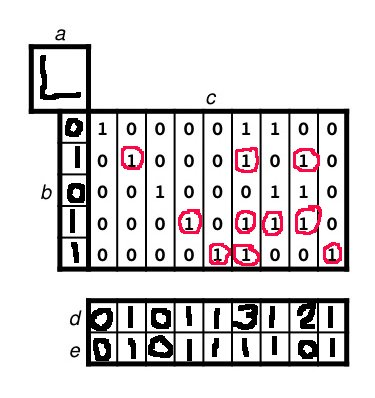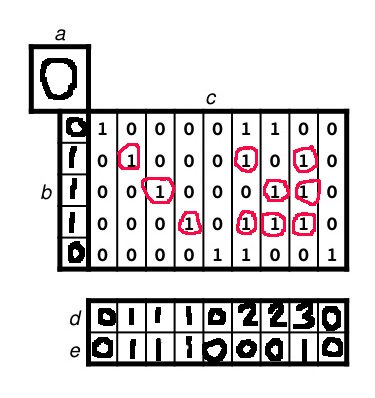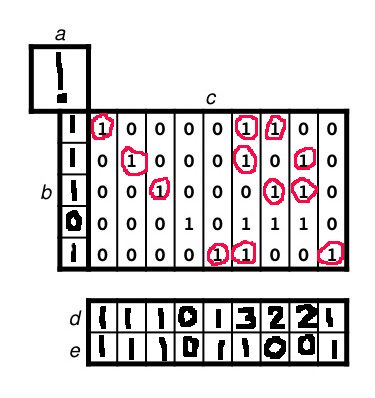Now it's your turn! Pick one of the driver licenses that you figured out was forged. You will encode the first four letters of the first name on the license. Don't forget that you need to use the Conversion Table and the encoders.

Next, ask a friend to act the part of the office prankster. S/he should change one bit from each code word (either make a 0 into a 1, or a 1 into a 0). Then, ask the prankster to pass this version of the codeword to your partner. The decoding process is very similar to encoding. It is important to use the decoder sheets (and not the encoders) at this stage.

• Write down the codeword from top to bottom in column (f).
• Each time there is a 1 in column (f), circle all the 1s in the row beside it.
• In each cell of row (h), write the number of circled 1s above it.
• In each cell of row (i), write 0 if the number above it is even (or zero), and 1 if it is odd.
• Find the error code corresponding to row (i) in the Syndrome/Error Table and write the error code in (j).
• Re-write the received codeword in row (k) (below (j)).
• If the cell in (j) is 0, write the number from (k) below it in (l). If the cell in (j) is 1, write the opposite number from that of (k) below it in (l).
• Using the first five digits of (l) as your code, look in the Conversion Table to get the corresponding alphabet letter.

Here is what the decoders would look like for the code
001100001  101000110  010101101  010111001  010100010  111111001 :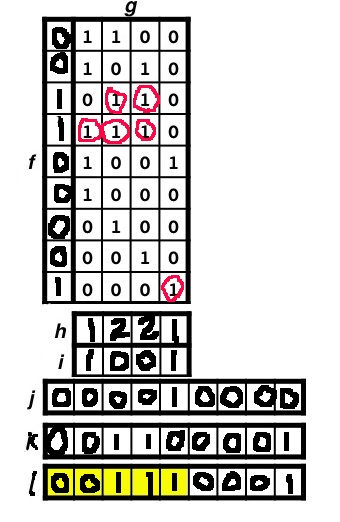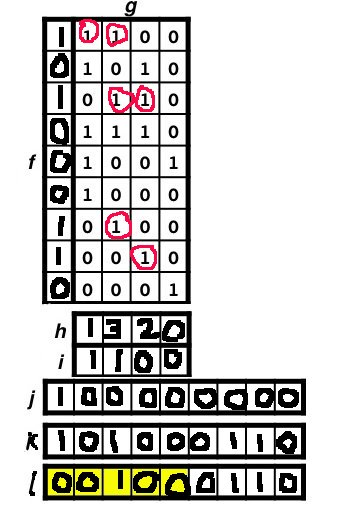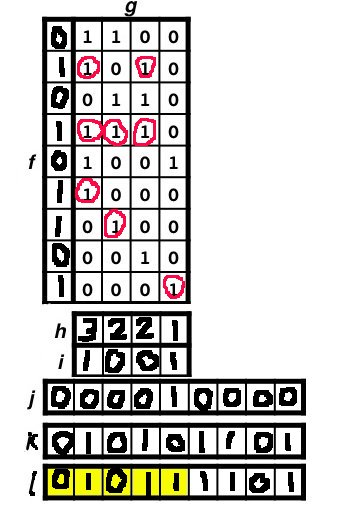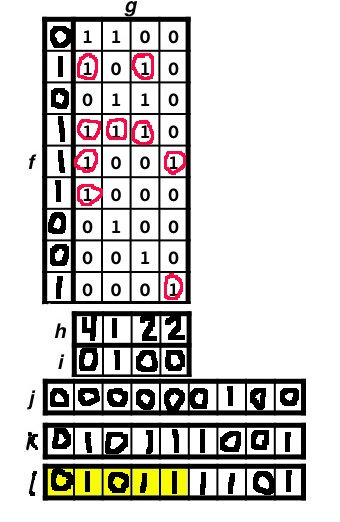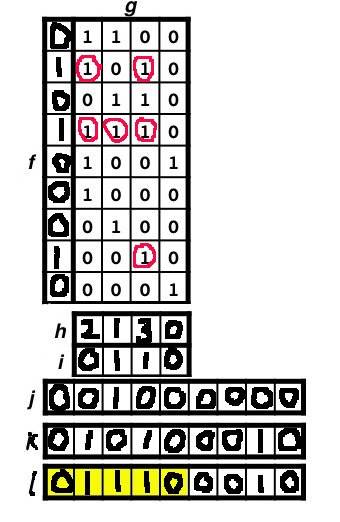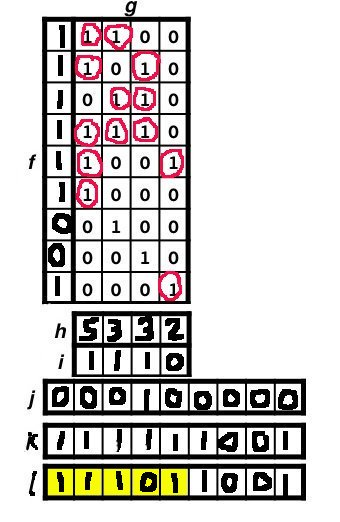If you look up the codes in the Conversion Table, you will notice that we have decoded the original message, even though there was an error in each codeword!

#### Authors

This workshop was created for Cornell's 4-H Career Explorations conference 2007 by Megan Owen, Saul Blanco-Rodriguez, and Lauren Childs.